Next: Bibliography Up: Nonlinear Switching State-Space Models Previous: Dirichlet distribution   Contents

# Probabilistic computations for MLP networks

In this appendix, it is shown how to evaluate the distribution of the outputs of an MLP network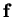, assuming the inputs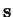and all the network weights are independent and Gaussian. These equations are needed in evaluating the ensemble learning cost function of the Bayesian NSSM in Section 6.2.

The exact model for our single-hidden-layer MLP networkis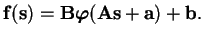(B.1)

The structure is shown in Figure B.1. The assumed distributions for all the parameters, which are assumed to be independent, are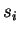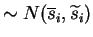(B.2)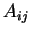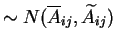(B.3)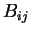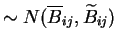(B.4)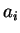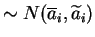(B.5)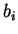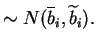(B.6)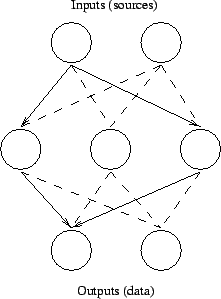The computations in the first layer of the network can be written as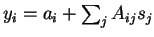. Since all the parameters involved are independent, the mean and the variance of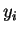are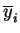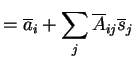(B.7)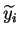(B.8)

Equation (B.8) follows from the identity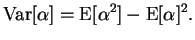(B.9)

The nonlinear activation function is handled with a truncated Taylor series approximation about the meanof the inputs. Using a second order approximation for the mean and first order for the variance yields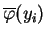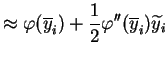(B.10)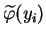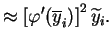(B.11)

The reason why these approximations are used is that they are the best ones that can be expressed in terms of the input mean and variance.

The computations of the output layer are given by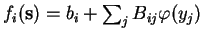. This may look the same as the one for the first layer, but there is the big difference that the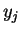are no longer independent. Their dependence does not, however, affect the evaluation of the mean of the outputs, which is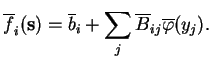(B.12)

The dependence betweenarises from the fact that eachmay potentially affect all of them. Hence, the variances ofwould be taken into the account incorrectly ifwere assumed independent.

Two possibly interfering paths can be seen in Figure B.1. Let us assume that the net weight of the left path is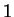and the weight of the right path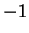, so that the two paths cancel each other out. If, however, the outputs of the hidden layer are incorrectly assumed to be independent, the estimated variance of the output will be greater than zero. The same effect can also happen the other way round when constructive inference of the two paths leads to underestimated output variance.

The effects of different components of the inputs on the outputs can be measured using the Jacobian matrix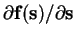of the mappingwith elements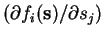. This leads to the approximation for the output variance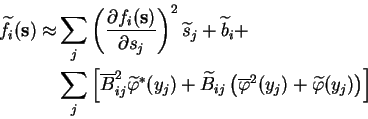(B.13)

where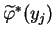denotes the posterior variance of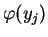without the contribution of the input variance. It can be computed as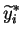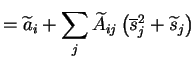(B.14)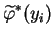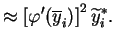(B.15)

The needed partial derivatives can be evaluated efficiently at the mean of the inputs with the chain rule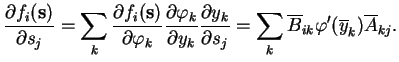(B.16)Next: Bibliography Up: Nonlinear Switching State-Space Models Previous: Dirichlet distribution   Contents
Antti Honkela 2001-05-30